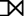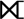## Relational Algebra

RELATIONAL ALGEBRA is a widely used procedural query language. It collects instances of relations as input and gives occurrences of relations as output. It uses various operations to perform this action. SQL Relational algebra query operations are performed recursively on a relation. The output of these operations is a new relation, which might be formed from one or more input relations.

In this tutorial, you will learn:

## Basic SQL Relational Algebra Operations

Relational Algebra devided in various groups

### Unary Relational Operations

• SELECT (symbol: σ)
• PROJECT (symbol: π)
• RENAME (symbol: ρ)

### Relational Algebra Operations From Set Theory

• UNION (υ)
• INTERSECTION ( ),
• DIFFERENCE (-)
• CARTESIAN PRODUCT ( x )

### Binary Relational Operations

• JOIN
• DIVISION

Let's study them in detail with solutions:

## SELECT (σ)

The SELECT operation is used for selecting a subset of the tuples according to a given selection condition. Sigma(σ)Symbol denotes it. It is used as an expression to choose tuples which meet the selection condition. Select operator selects tuples that satisfy a given predicate.

`σp(r)`

`σ` is the predicate

`r` stands for relation which is the name of the table

`p` is prepositional logic

Example 1

```σ topic = "Database" (Tutorials)
```

Output - Selects tuples from Tutorials where topic = 'Database'.

Example 2

```σ topic = "Database" and author = "guru99"( Tutorials)
```

Output - Selects tuples from Tutorials where the topic is 'Database' and 'author' is guru99.

Example 3

```σ sales > 50000 (Customers)
```

Output - Selects tuples from Customers where sales is greater than 50000

## Projection(π)

The projection eliminates all attributes of the input relation but those mentioned in the projection list. The projection method defines a relation that contains a vertical subset of Relation.

This helps to extract the values of specified attributes to eliminates duplicate values. (pi) symbol is used to choose attributes from a relation. This operator helps you to keep specific columns from a relation and discards the other columns.

Example of Projection:

Consider the following table

CustomerID CustomerName Status
2 Amazon Active
3 Apple Inactive
4 Alibaba Active

Here, the projection of CustomerName and status will give

```Π CustomerName, Status (Customers)
```
CustomerName Status
Amazon Active
Apple Inactive
Alibaba Active

## Rename (ρ)

Rename is a unary operation used for renaming attributes of a relation.

ρ (a/b)R will rename the attribute 'b' of relation by 'a'.

## Union operation (υ)

UNION is symbolized by ∪ symbol. It includes all tuples that are in tables A or in B. It also eliminates duplicate tuples. So, set A UNION set B would be expressed as:

The result <- A ∪ B

For a union operation to be valid, the following conditions must hold -

• R and S must be the same number of attributes.
• Attribute domains need to be compatible.
• Duplicate tuples should be automatically removed.

Example

Consider the following tables.

column 1 column 2 column 1 Table A Table B 1 1 1 1 1 2 1 3

A ∪ B gives

column 1 column 2 Table A ∪ B 1 1 1 2 1 3

## Set Difference (-)

- Symbol denotes it. The result of A - B, is a relation which includes all tuples that are in A but not in B.

• The attribute name of A has to match with the attribute name in B.
• The two-operand relations A and B should be either compatible or Union compatible.
• It should be defined relation consisting of the tuples that are in relation A, but not in B.

Example

```A-B
```
column 1 column 2 Table A - B 1 2

## Intersection

An intersection is defined by the symbol ∩

A ∩ B

Defines a relation consisting of a set of all tuple that are in both A and B. However, A and B must be union-compatible.

Example:

```A ∩ B
```
column 1 column 2 Table A ∩ B 1 1

## Cartesian Product(X) in DBMS

Cartesian Product in DBMS is an operation used to merge columns from two relations. Generally, a cartesian product is never a meaningful operation when it performs alone. However, it becomes meaningful when it is followed by other operations. It is also called Cross Product or Cross Join.

Example – Cartesian product

σ column 2 = '1' (A X B)

Output – The above example shows all rows from relation A and B whose column 2 has value 1

column 1 column 2 σ column 2 = '1' (A X B) 1 1 1 1

## Join Operations

Join operation is essentially a cartesian product followed by a selection criterion.

Join operation denoted by ⋈.

JOIN operation also allows joining variously related tuples from different relations.

Types of JOIN:

Various forms of join operation are:

Inner Joins:

• Theta join
• EQUI join
• Natural join

Outer join:

• Left Outer Join
• Right Outer Join
• Full Outer Join

## Inner Join:

In an inner join, only those tuples that satisfy the matching criteria are included, while the rest are excluded. Let's study various types of Inner Joins:

## Theta Join:

The general case of JOIN operation is called a Theta join. It is denoted by symbol θ

Example

`A ⋈θ B`

Theta join can use any conditions in the selection criteria.

For example:

```A ⋈ A.column 2 >  B.column 2 (B)
```
column 1 column 2 A ⋈ A.column 2 > B.column 2 (B) 1 2

## EQUI join:

When a theta join uses only equivalence condition, it becomes a equi join.

For example:

```A ⋈ A.column 2 =  B.column 2 (B)
```
column 1 column 2 A ⋈ A.column 2 = B.column 2 (B) 1 1

EQUI join is the most difficult operations to implement efficiently using SQL in an RDBMS and one reason why RDBMS have essential performance problems.

## NATURAL JOIN (⋈)

Natural join can only be performed if there is a common attribute (column) between the relations. The name and type of the attribute must be same.

Example

Consider the following two tables

Num Square C 2 4 3 9
Num Cube D 2 8 3 27
```C ⋈ D
```
Num Square Cube C ⋈ D 2 4 4 3 9 27

## OUTER JOIN

In an outer join, along with tuples that satisfy the matching criteria, we also include some or all tuples that do not match the criteria.

## Left Outer Join(AB)

In the left outer join, operation allows keeping all tuple in the left relation. However, if there is no matching tuple is found in right relation, then the attributes of right relation in the join result are filled with null values.

Consider the following 2 Tables

Num Square A 2 4 3 9 4 16
Num Cube B 2 8 3 18 5 75
```AB
```
Num Square Cube A ⋈ B 2 4 4 3 9 9 4 16 -

## Right Outer Join: ( AB )

In the right outer join, operation allows keeping all tuple in the right relation. However, if there is no matching tuple is found in the left relation, then the attributes of the left relation in the join result are filled with null values.

```AB
```
Num Cube Square A ⋈ B 2 8 4 3 18 9 5 75 -

## Full Outer Join: ( AB)

In a full outer join, all tuples from both relations are included in the result, irrespective of the matching condition.

```AB
```
Num Cube Square A ⋈ B 2 4 8 3 9 18 4 16 - 5 - 75

## Summary

Operation(Symbols)

Purpose

Select(σ)

The SELECT operation is used for selecting a subset of the tuples according to a given selection condition

Projection(π)

The projection eliminates all attributes of the input relation but those mentioned in the projection list.

Union Operation(∪)

UNION is symbolized by symbol. It includes all tuples that are in tables A or in B.

Set Difference(-)

- Symbol denotes it. The result of A - B, is a relation which includes all tuples that are in A but not in B.

Intersection(∩)

Intersection defines a relation consisting of a set of all tuple that are in both A and B.

Cartesian Product(X)

Cartesian operation is helpful to merge columns from two relations.

Inner Join

Inner join, includes only those tuples that satisfy the matching criteria.

Theta Join(θ)

The general case of JOIN operation is called a Theta join. It is denoted by symbol θ.

EQUI Join

When a theta join uses only equivalence condition, it becomes a equi join.

Natural Join(⋈)

Natural join can only be performed if there is a common attribute (column) between the relations.

Outer Join

In an outer join, along with tuples that satisfy the matching criteria.

In the left outer join, operation allows keeping all tuple in the left relation.

Right Outer join()

In the right outer join, operation allows keeping all tuple in the right relation.

In a full outer join, all tuples from both relations are included in the result irrespective of the matching condition.

YOU MIGHT LIKE: